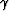# Wave-equation angle-domain common-image gathers for converted waves: Part 2

Daniel A. Rosales, Sergey Fomel, Biondo L. Biondi, and Paul C. Sava

daniel@sep.stanford.edu, sergey.fomel@beg.utexas.edu, biondo@sep.stanford.edu, sava.paul@beg.utexas.edu

# ABSTRACT

Common-image gathers are very useful for velocity and amplitude analysis. Wavefield-extrapolation methods produce Angle-Domain Common-Image Gathers (ADCIGs). For the conventional PP case, ADCIGs are a function of the opening angle. However, the ADCIGs for converted-wave data (PS-ADCIGs) are a function of the half-aperture angle, that is, the incidence angle plus the reflection angle. In PS-ADCIGs, both the P-to-S velocity ratio () and the image dip play a major role in transforming the subsurface offset into the opening angle. We introduce a simple methodology to compute PS-ADCIGs. Our methodology exploits the robustness of computing PP-ADCIGs, and incorporates the velocity ratio (), with an image dip field, which is estimated along the prestack image. Our methodology also transforms the half-aperture angle in PS-ADCIGs into an independent P-incidence angle to form P-ADCIGs, and an independent S-reflection angle to form S-ADCIGs. Numerical studies show that when the P-to-S velocity ratio and image midpoint information are not incorporated, the error in computing PS-ADCIGs is large enough to introduce artifacts in the velocity model. Synthetic results show the accuracy of the transformation introduced in this paper. Real data results on the 2-D Mahogany field show the practical application and implications for converted-wave angle-domain common-image gathers.